Successfully reported this slideshow.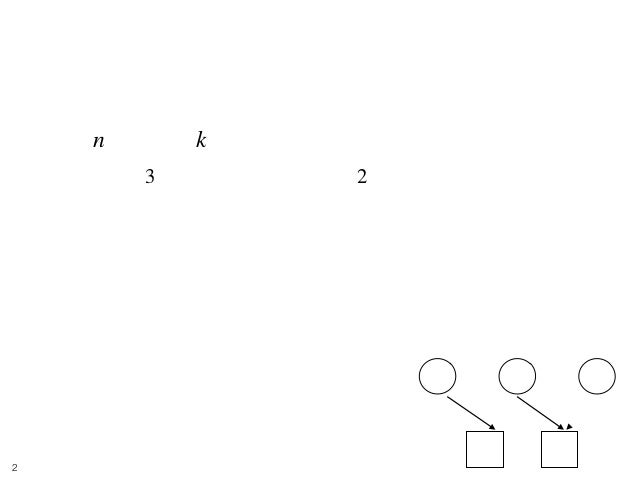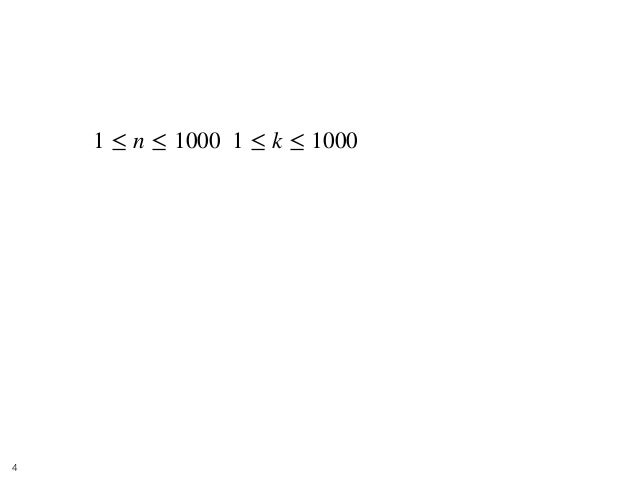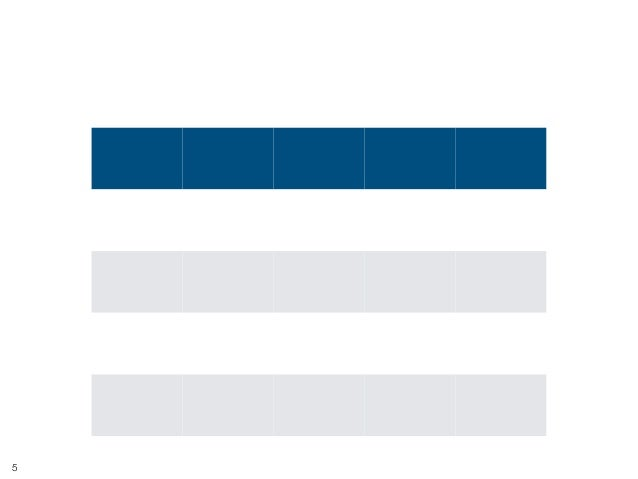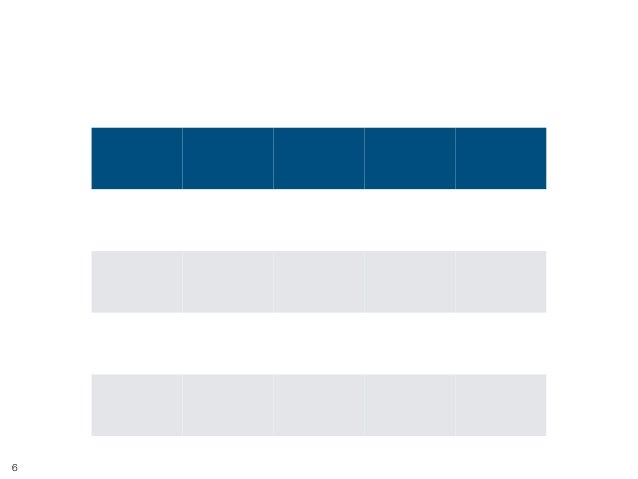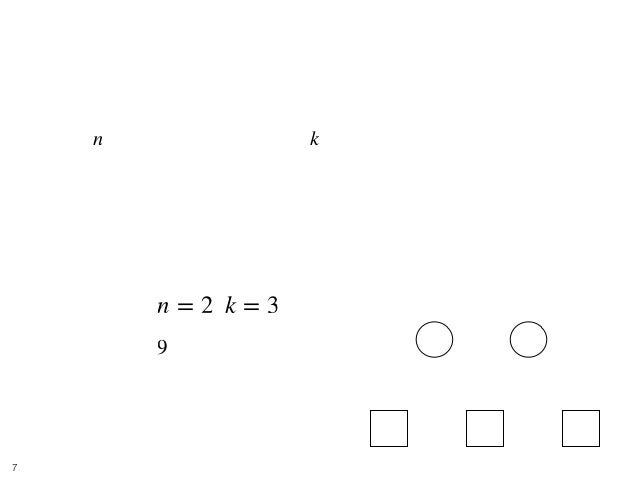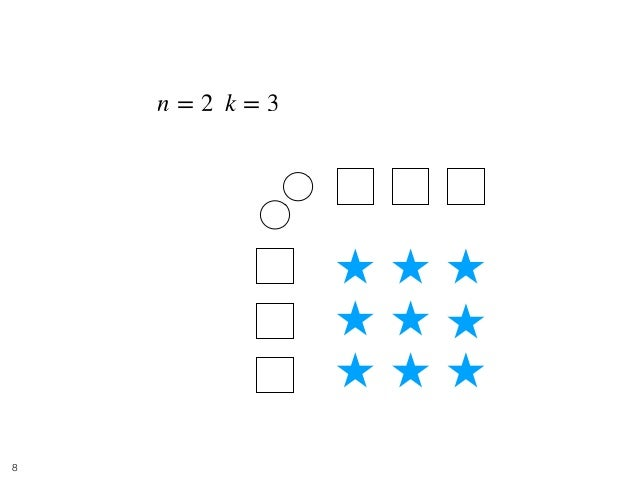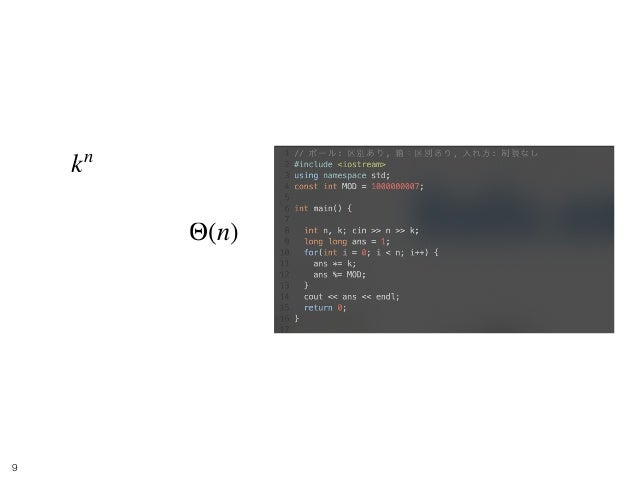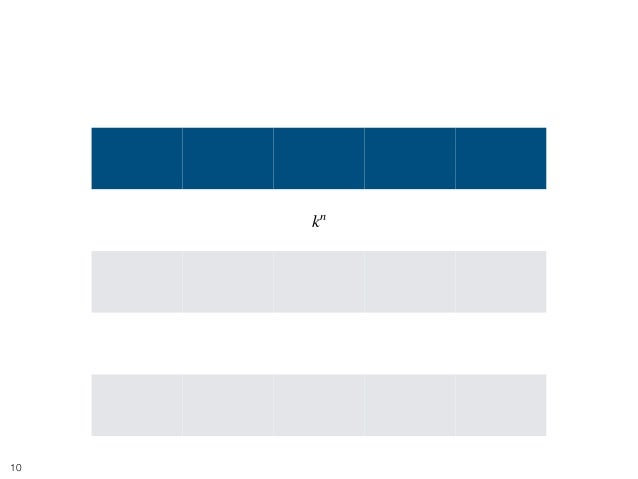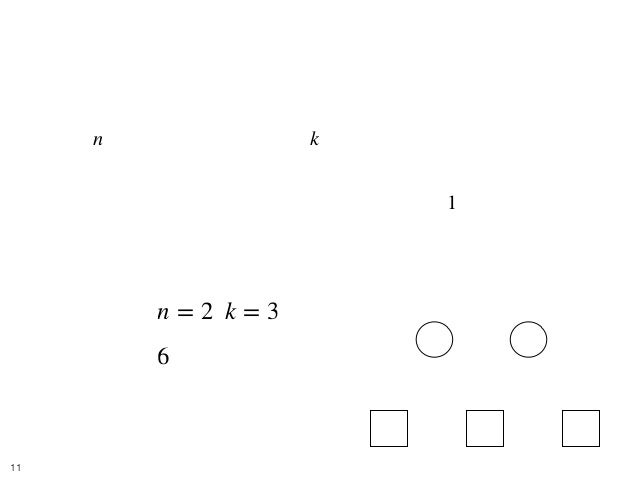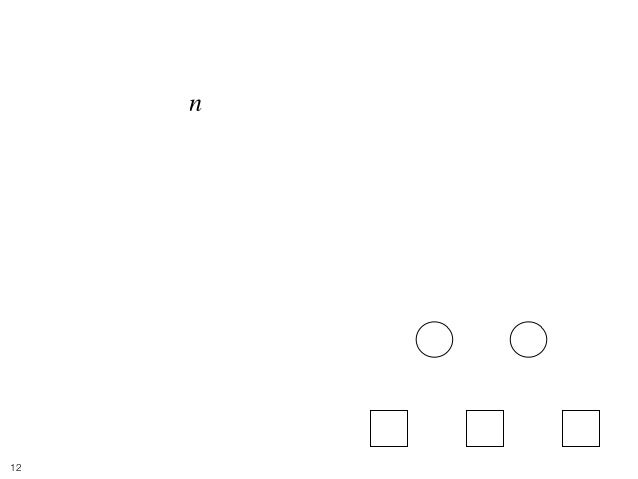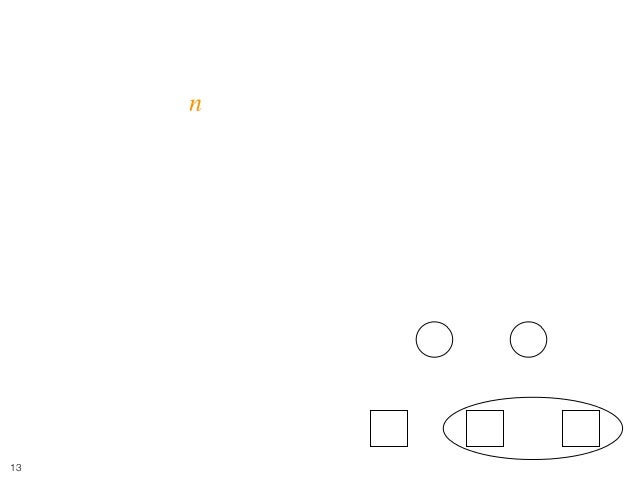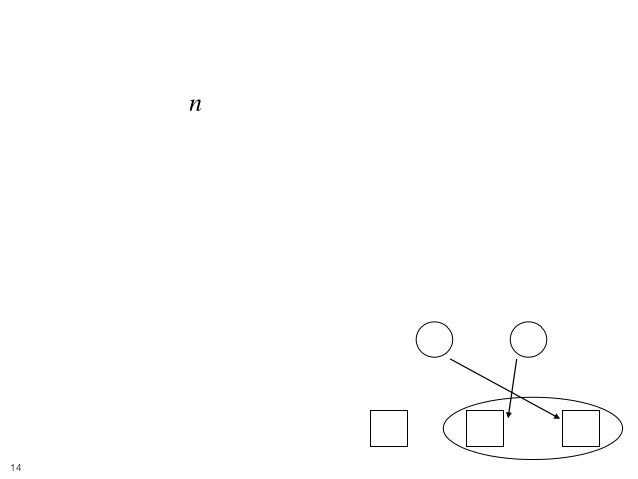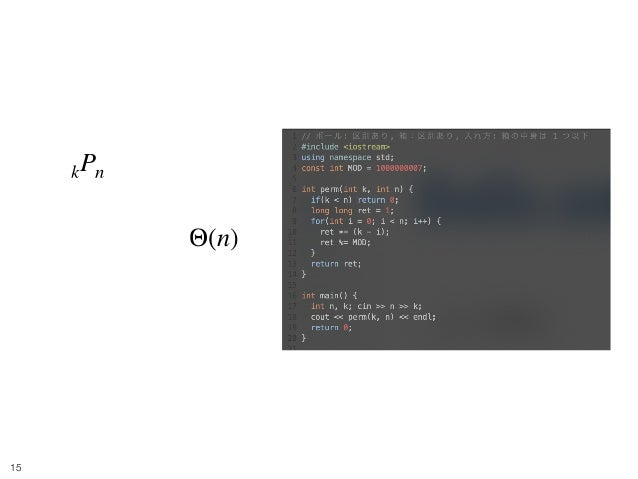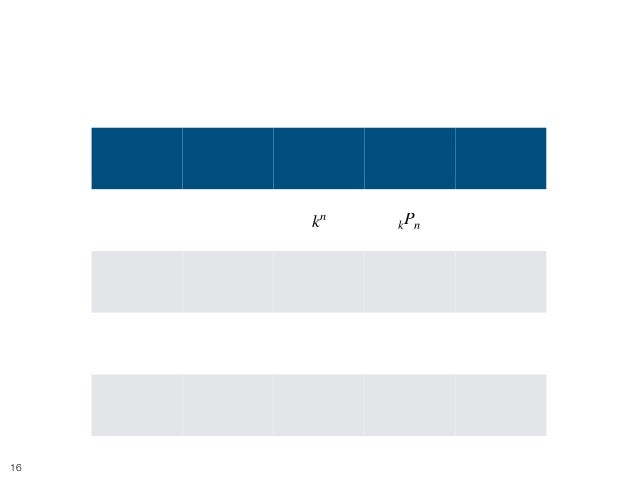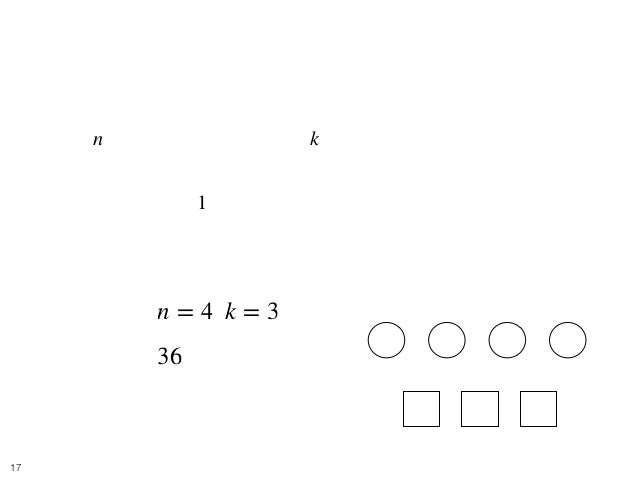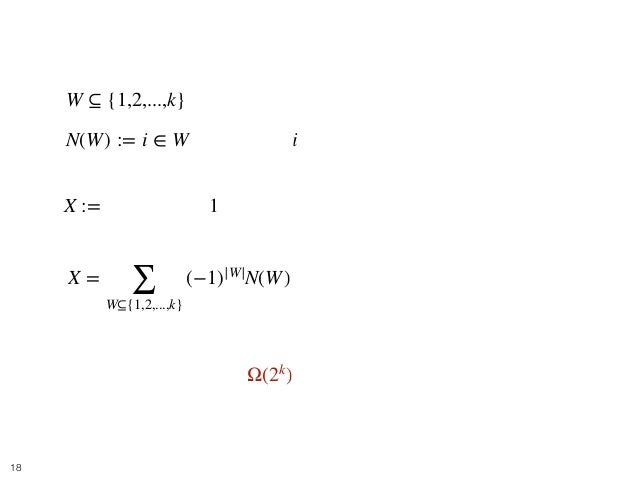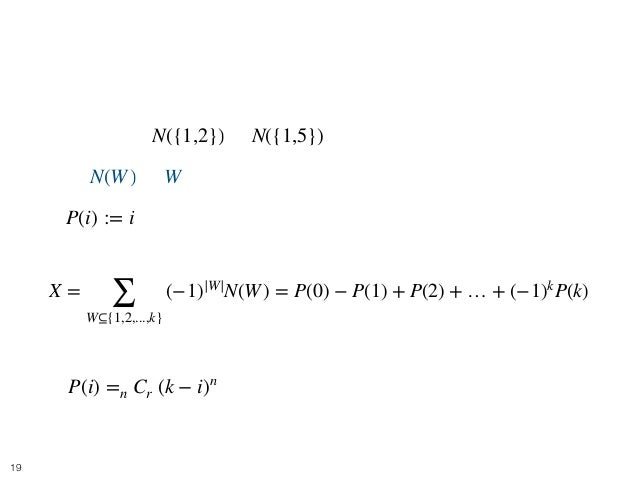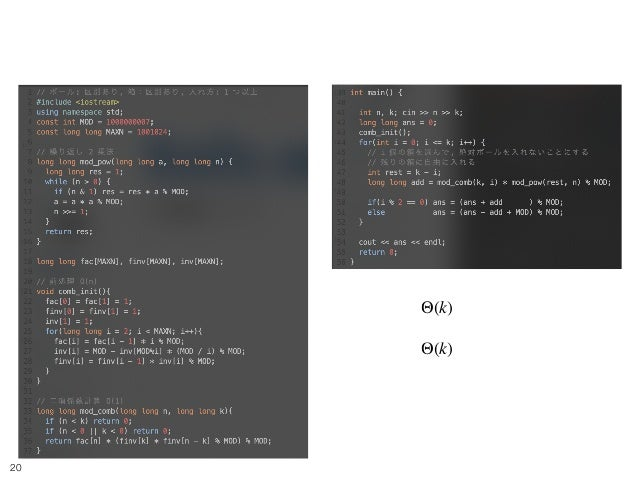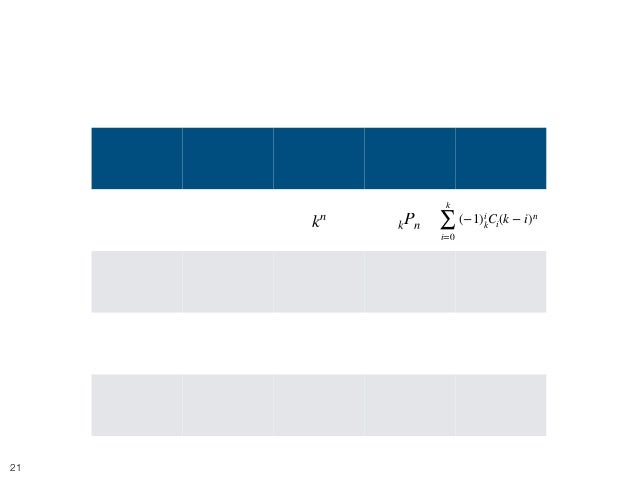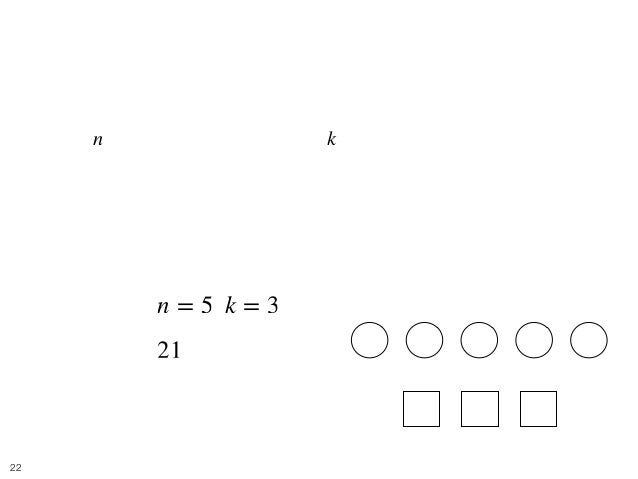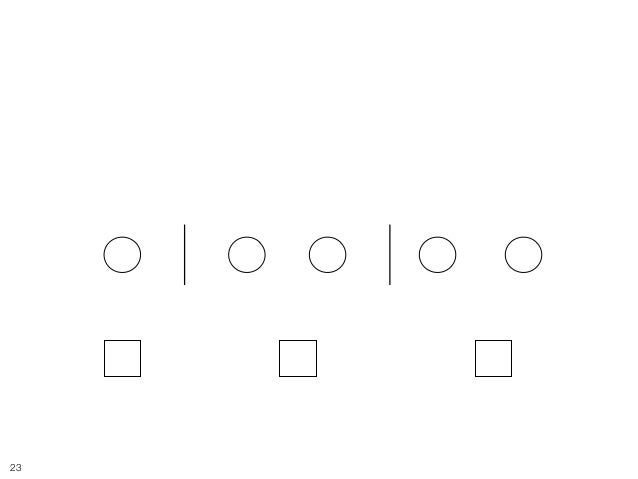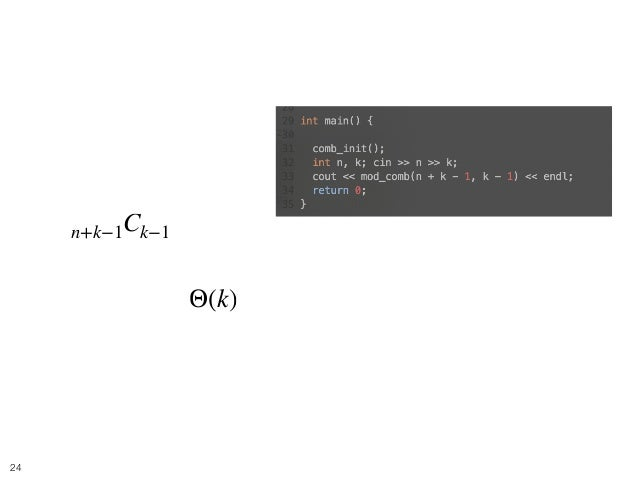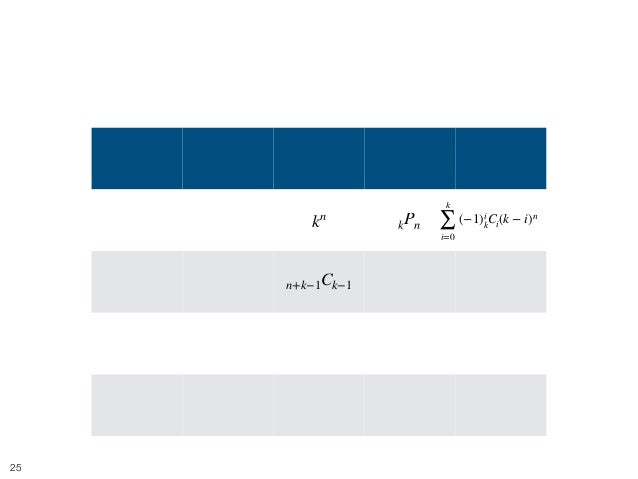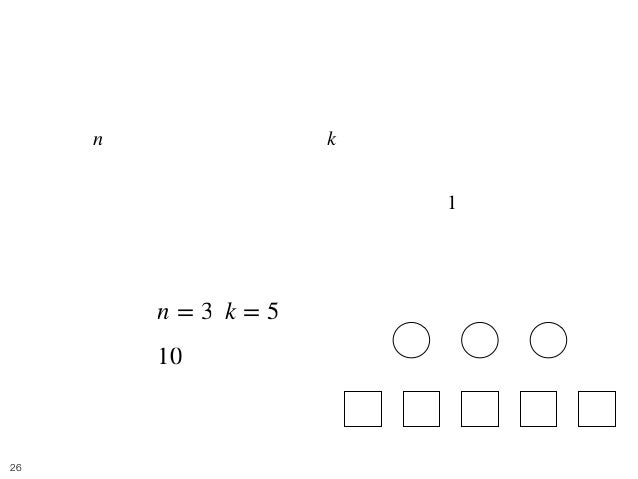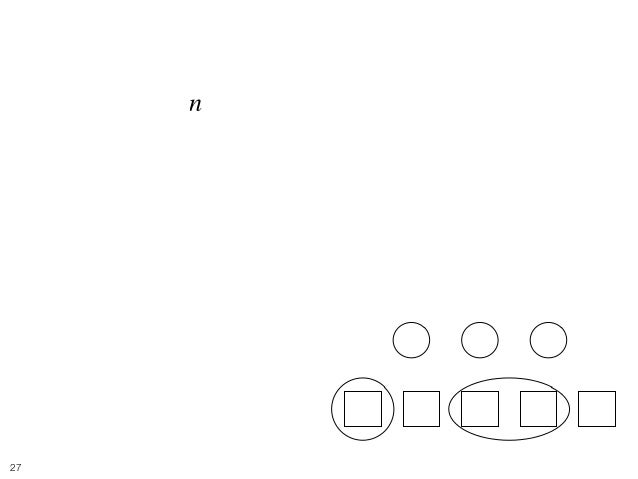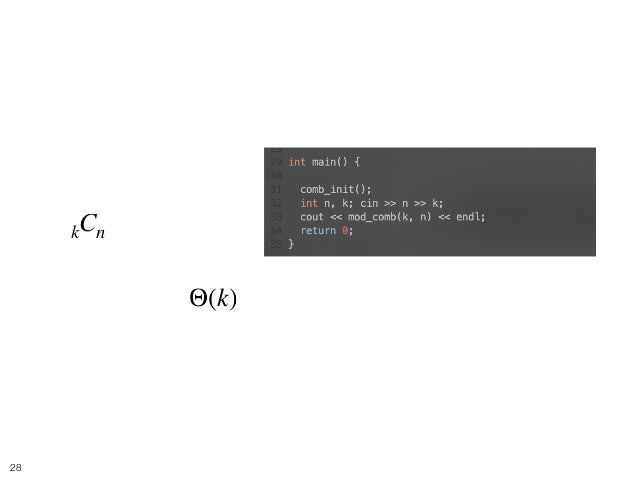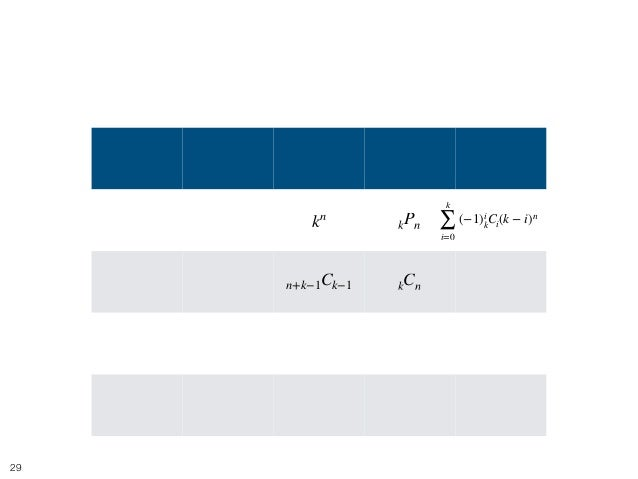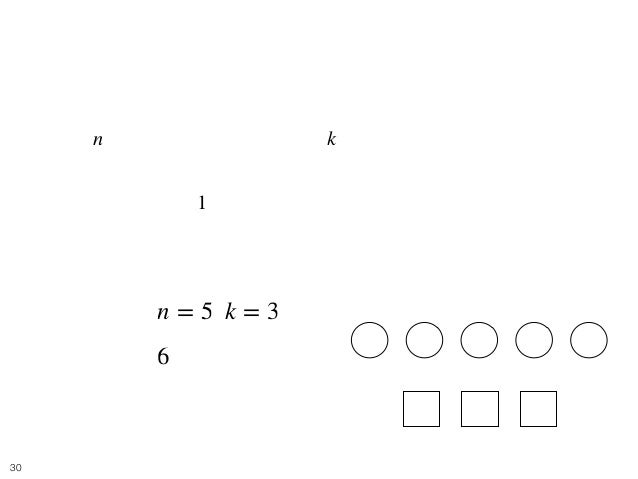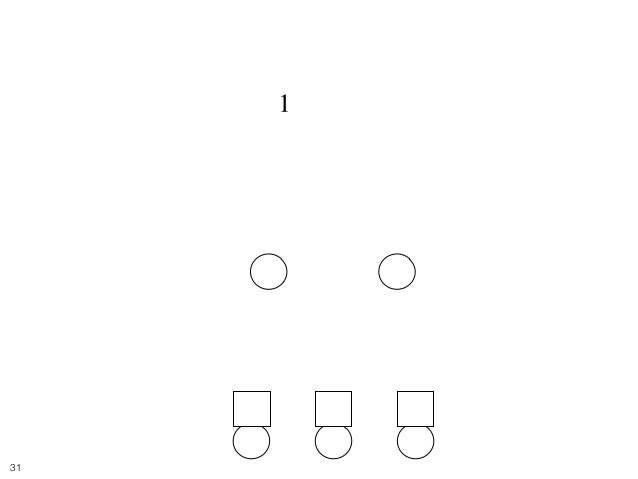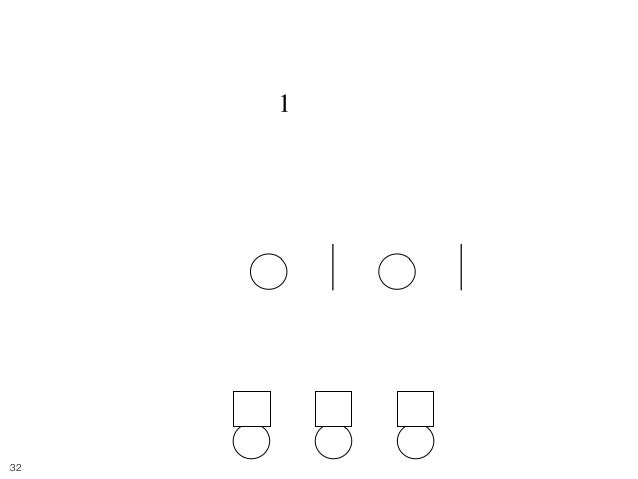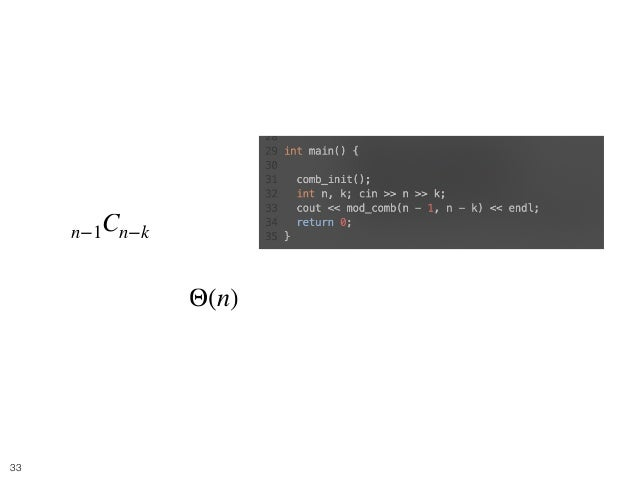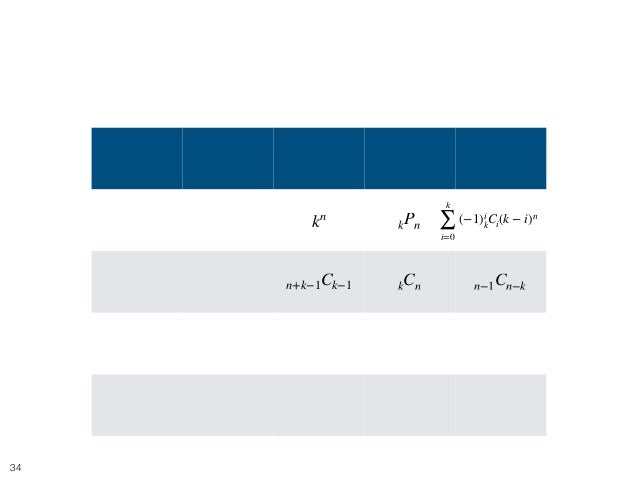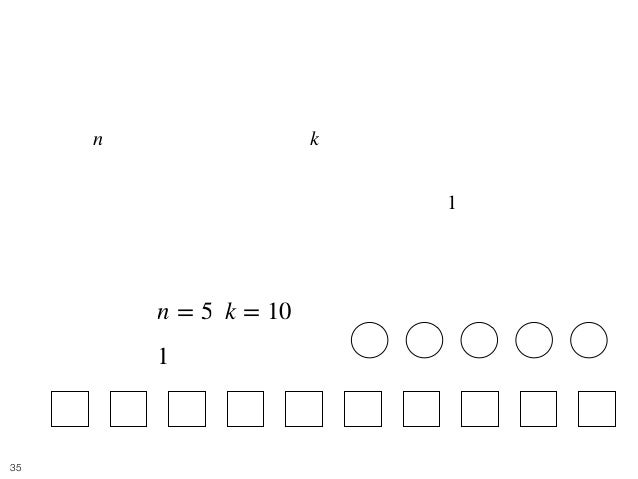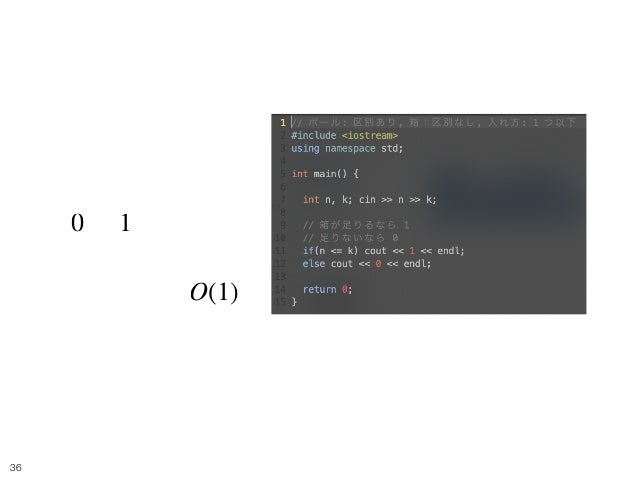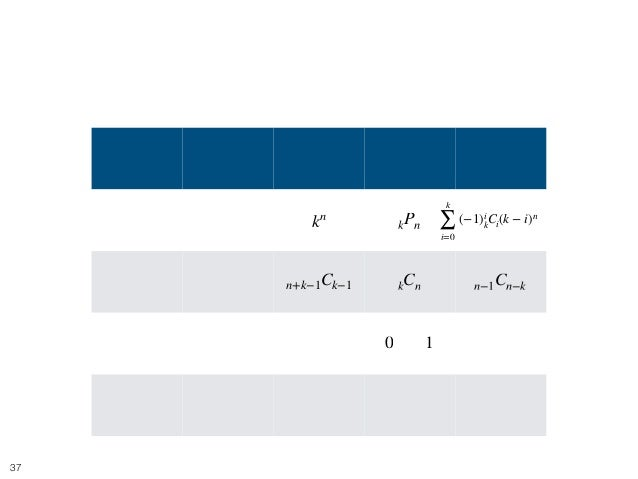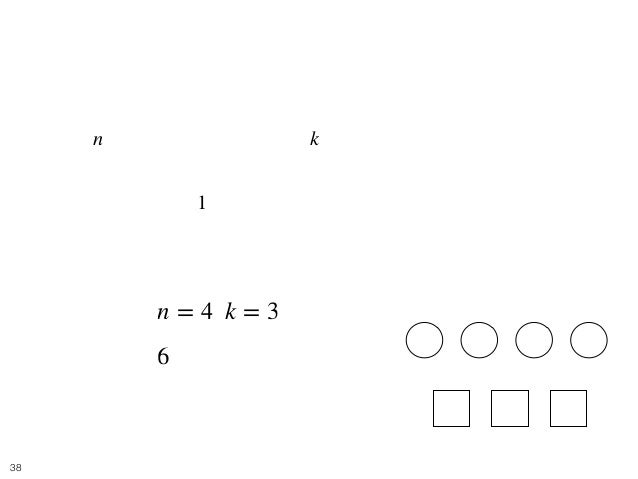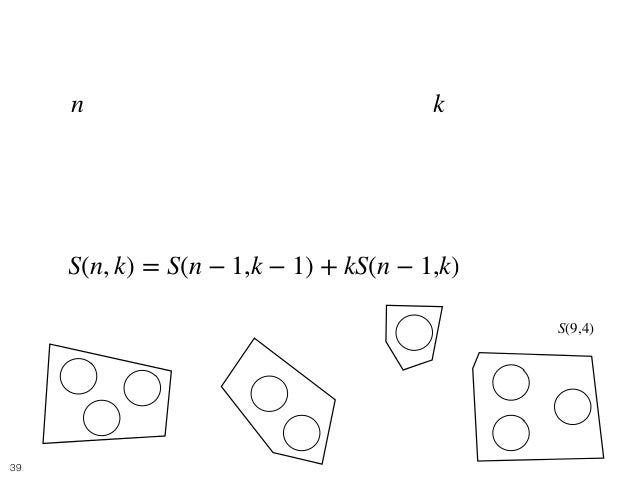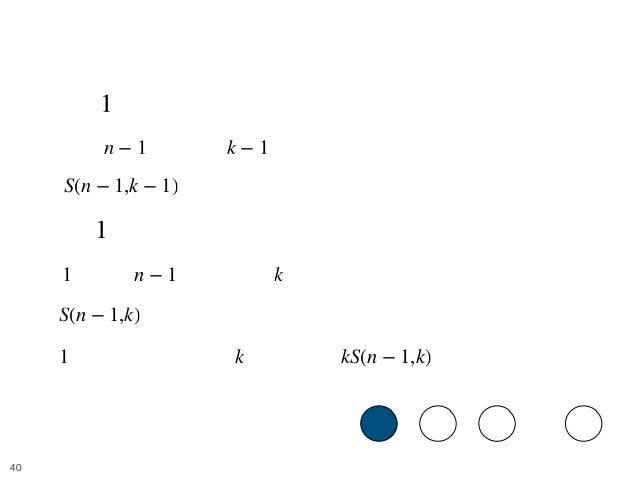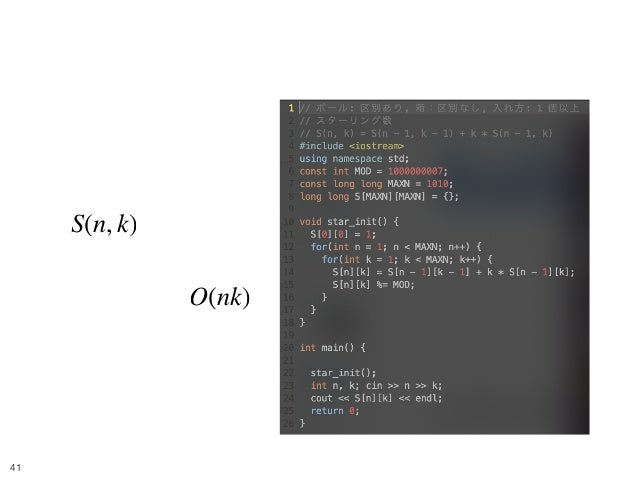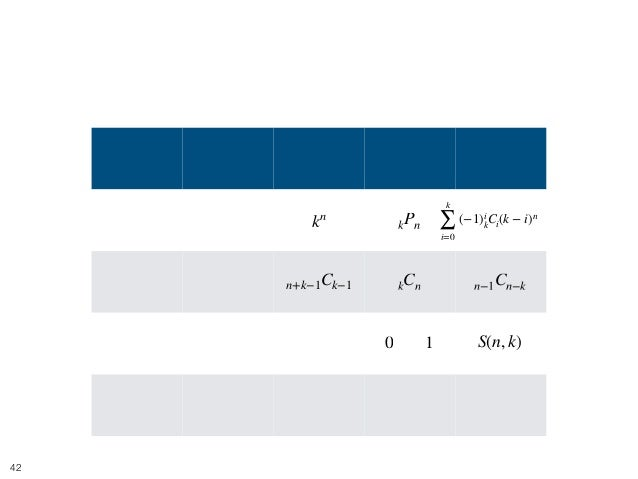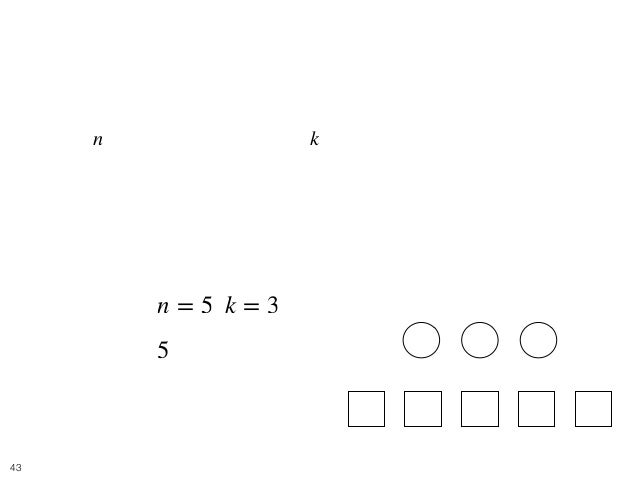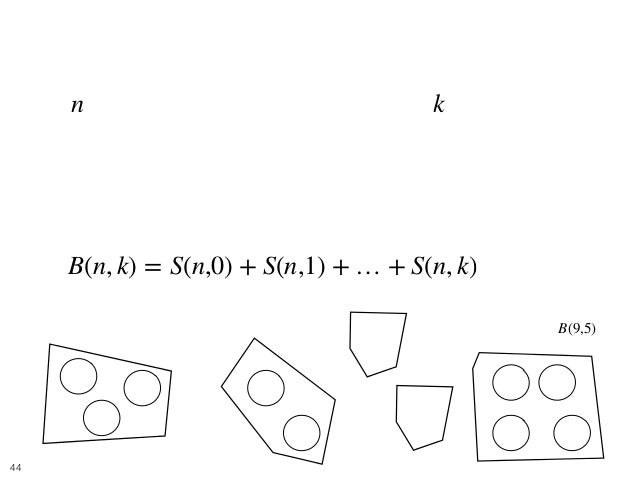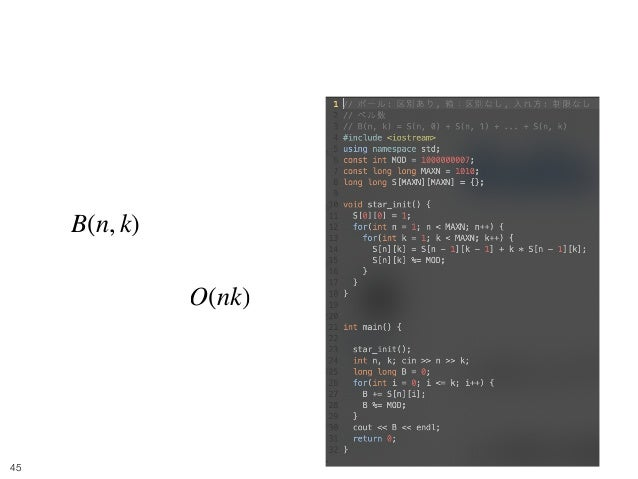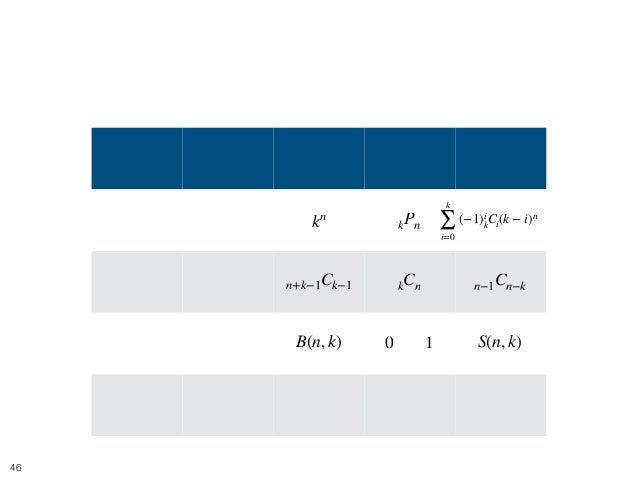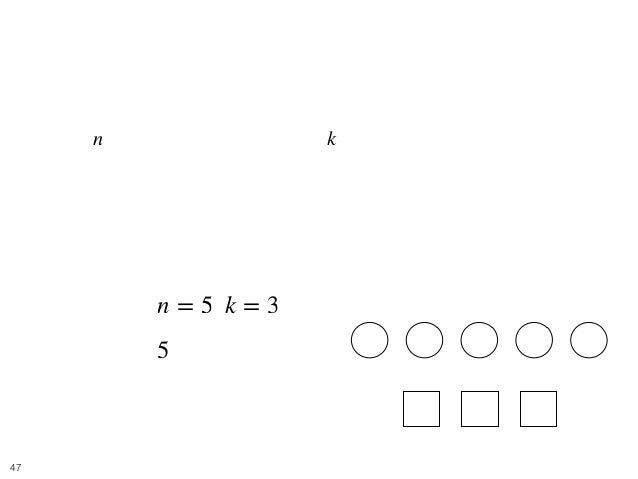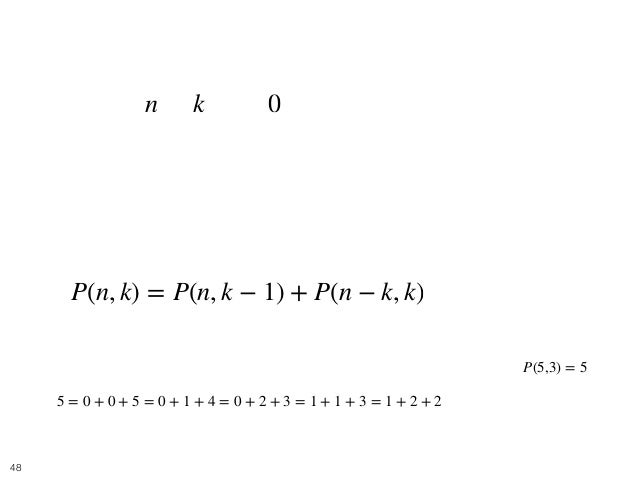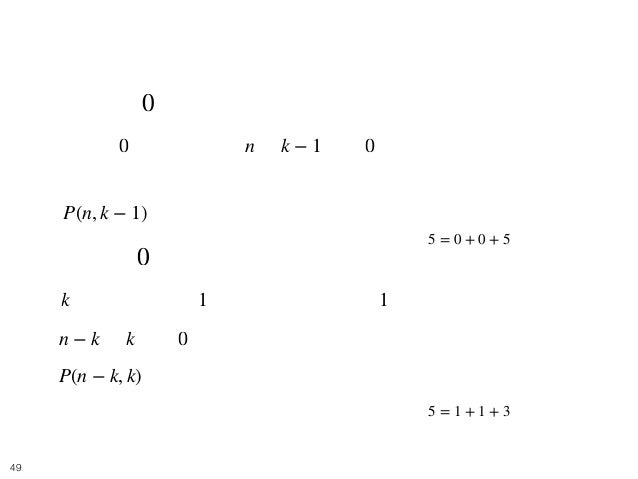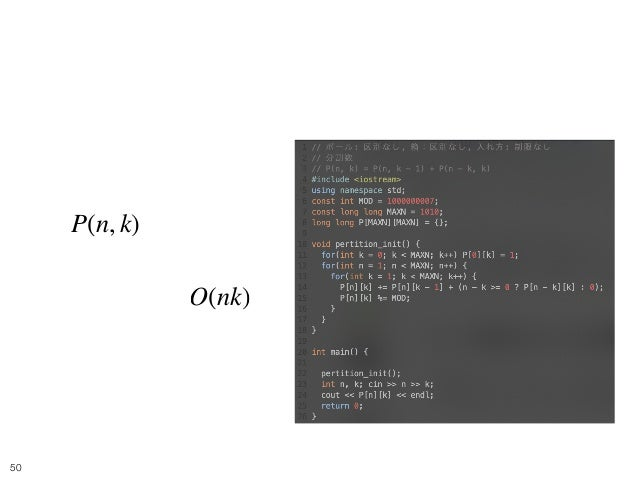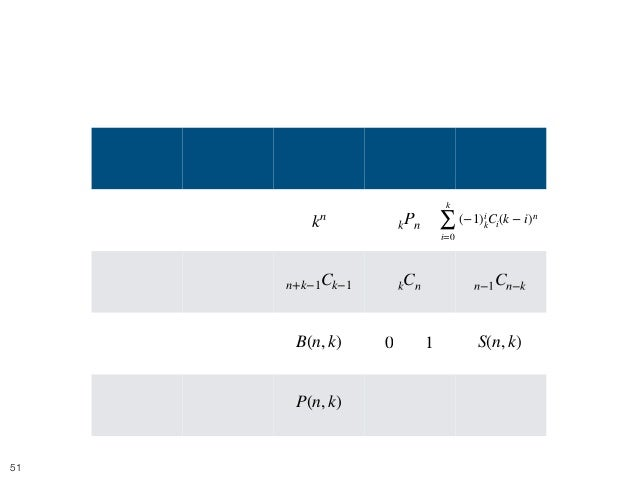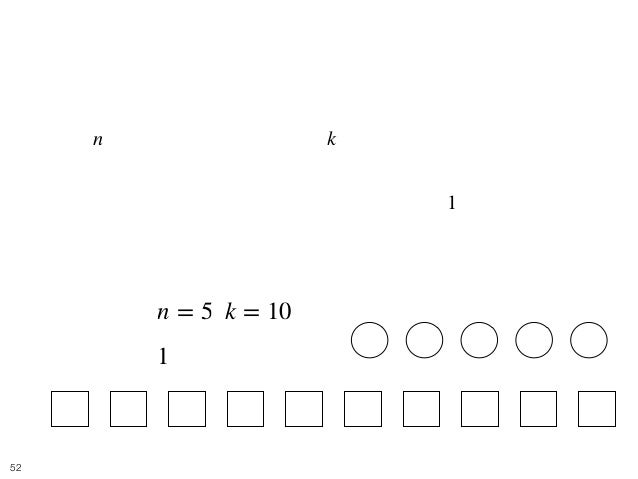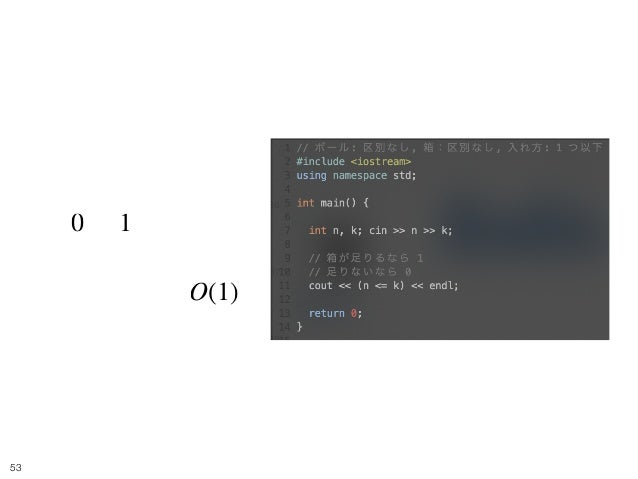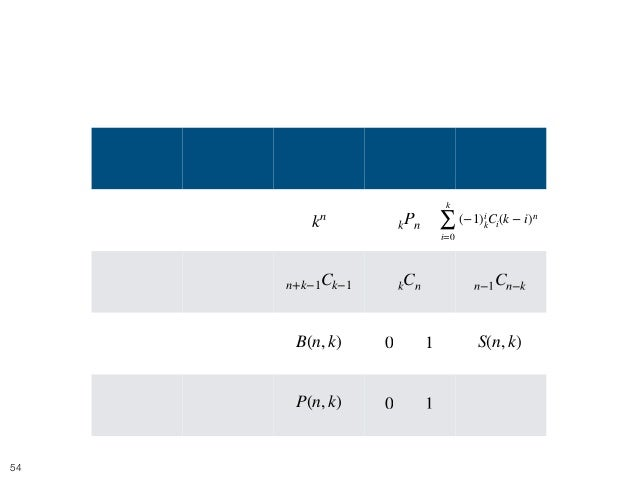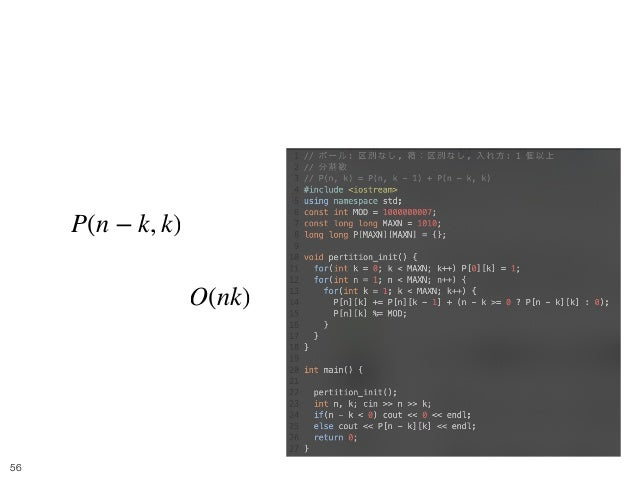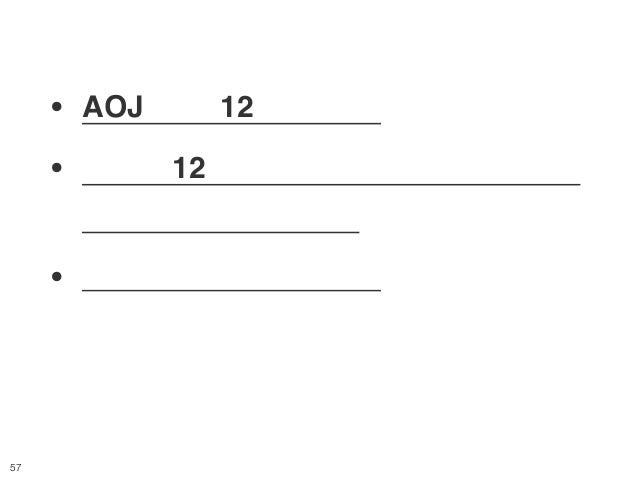Upcoming SlideShare
×

# 写像 12 相

Oct 24, 2019 北海道大学競技プログラミングサークル勉強会
※文字が見えない場合は、ダウンロードするかフルスクリーンにしてご覧ください

• Full Name
Comment goes here.

Are you sure you want to Yes No• Be the first to comment

### 写像 12 相

1. 1. 写像 12 相を題材にした, 基本的な数え上げ問題に対す るアプローチ Hokkaido Univ. monkukui
2. 2. 写像 12 相とは • 写像 12 相: • 個の玉を 個の箱に入れる問題の総称 • e.g. 個の区別できるボールを 個の区別できる箱へ入れる ときの通り数は？？ • どうして写像と呼ばれるか • 写像: 集合の各元を、他の集合の元のいずれかへ対応づけること •ボールの箱への割り当てと考えると, これは写像 n k 3 2 !2 Ba Ba Ba Bo Bo
3. 3. 達成目標 • 写像 12 相を題材に, 基本的な数え上げ問題へのア プローチを考える • AtCoder のレートが上がることが見込める !3
4. 4. 準備 • 制約は以下で統一されているものとする • ,1 ≤ n ≤ 1000 1 ≤ k ≤ 1000 !4
5. 5. 目次 !5 玉 箱 制限なし 1 個以内 1 個以上 区別する 区別する 区別しない 区別する 区別する 区別しない 区別しない 区別しない
6. 6. 目次 !6 玉 箱 制限なし 1 個以内 1 個以上 区別する 区別する ここ 区別しない 区別する 区別する 区別しない 区別しない 区別しない
7. 7. Balls and Boxes 1 • [問題] 難易度: 茶 • 個の区別できるボールを, 個の区別できる箱に入れるとき、 可能な入れ方の総数を求めよ. • ただし、箱に対する制限はないものとする. • e.g. • [入力] , • [出力] n k n = 2 k = 3 9 !7 Ba Ba Bo Bo Bo
8. 8. Balls and Boxes 1 • [入力] ,n = 2 k = 3 !8 1 2 1 2 3 1 2 3
9. 9. Balls and Boxes 1 • が答え • 時間計算量 kn Θ(n) !9
10. 10. 目次 !10 玉 箱 制限なし 1 個以内 1 個以上 区別する 区別する ここ 区別しない 区別する 区別する 区別しない 区別しない 区別しない kn
11. 11. Balls and Boxes 2 • [問題] 難易度: 茶 • 個の区別できるボールを, 個の区別できる箱に入れるとき、 可能な入れ方の総数を求めよ. • 各箱に入れることのできるボールの数は高々 個とする. • e.g. • [入力] , • [出力] n k 1 n = 2 k = 3 6 !11 Ba Ba Bo Bo Bo
12. 12. Balls and Boxes 2 • 入れる箱を 個選んで • ボールを入れる n !12 Ba Ba Bo Bo Bo
13. 13. Balls and Boxes 2 • 入れる箱を 個選んで • ボールを入れる n !13 Ba Ba Bo Bo Bo
14. 14. Balls and Boxes 2 • 入れる箱を 個選んで • ボールを入れる n !14 Ba Ba Bo Bo Bo
15. 15. Balls and Boxes 2 • が答え • 時間計算量 kPn Θ(n) !15
16. 16. 目次 !16 玉 箱 制限なし 1 個以内 1 個以上 区別する 区別する ここ 区別しない 区別する 区別する 区別しない 区別しない 区別しない kn kPn
17. 17. Balls and Boxes 3 • [問題] 難易度: 青 • 個の区別できるボールを, 個の区別できる箱に入れるとき、 可能な入れ方の総数を求めよ. • どの箱にも、 つ以上のボールを入れる必要がある. • e.g. • [入力] , • [出力] n k 1 n = 4 k = 3 36 !17 Ba Ba Ba Ba Bo Bo Bo
18. 18. Balls and Boxes 3 • とする. • に対して, 箱 にボールが入らないような入れ方の 総数. • どの箱にも、 つ以上のボールを入れる入れ方の総数. • 包除原理より, となる. これを愚直に計算すると, かかってしまう. W ⊆ {1,2,...,k} N(W) := i ∈ W i X := 1 X = ∑ W⊆{1,2,...,k} (−1)|W| N(W) Ω(2k ) !18
19. 19. Balls and Boxes 3 • 対称性を利用する • 例えば, と は同じになる. • は の濃度にのみ依存した関数である. • 箇所の箱にボールが入らないような入れ方の総数 となる. • で計算可能 N({1,2}) N({1,5}) N(W) W P(i) := i X = ∑ W⊆{1,2,...,k} (−1)|W| N(W) = P(0) − P(1) + P(2) + … + (−1)k P(k) P(i) =n Cr (k − i)n !19
20. 20. Balls and Boxes 3 !20 前処理: Θ(k) 本処理: Θ(k) nCr や べき乗の処理は 以下の検索ワードでググりましょう (繰り返し 2 乗法, 逆元)
21. 21. 目次 !21 玉 箱 制限なし 1 個以内 1 個以上 区別する 区別する 区別しない 区別する ここ 区別する 区別しない 区別しない 区別しない kn kPn k ∑ i=0 (−1)i kCi(k − i)n
22. 22. Balls and Boxes 4 • [問題] 難易度: 緑 • 個の区別できないボールを, 個の区別できる箱に入れると き、可能な入れ方の総数を求めよ. • ただし、箱に対する制限はないものとする. • e.g. • [入力] , • [出力] n k n = 5 k = 3 21 !22 Ba Ba Ba Ba Bo Bo Bo Ba
23. 23. Balls and Boxes 4 • （区別できない）棒とボールの並び替えに帰着 !23 Ba Ba Ba Ba Bo Bo Bo Ba
24. 24. Balls and Boxes 4 • が答え • 時間計算量 n+k−1Ck−1 Θ(k) !24
25. 25. 目次 !25 玉 箱 制限なし 1 個以内 1 個以上 区別する 区別する 区別しない 区別する ここ 区別する 区別しない 区別しない 区別しない kn kPn n+k−1Ck−1 k ∑ i=0 (−1)i kCi(k − i)n
26. 26. Balls and Boxes 5 • [問題] 難易度: 茶 • 個の区別できないボールを, 個の区別できる箱に入れると き、可能な入れ方の総数を求めよ. • 各箱に入れることのできるボールの数は高々 個とする. • e.g. • [入力] , • [出力] n k 1 n = 3 k = 5 10 !26 Ba Ba Ba Bo Bo Bo BoBo
27. 27. Balls and Boxes 5 • 入れる箱を 個選べば良いn !27 Ba Ba Ba Bo Bo Bo BoBo
28. 28. Balls and Boxes 5 • が答え • 時間計算量 kCn Θ(k) !28
29. 29. 目次 !29 玉 箱 制限なし 1 個以内 1 個以上 区別する 区別する 区別しない 区別する まだ半分.. 区別する 区別しない 区別しない 区別しない kn kPn n+k−1Ck−1 kCn k ∑ i=0 (−1)i kCi(k − i)n
30. 30. Balls and Boxes 6 • [問題] 難易度: 茶 • 個の区別できないボールを, 個の区別できる箱に入れると き、可能な入れ方の総数を求めよ. • どの箱にも、 つ以上のボールを入れる必要がある. • e.g. • [入力] , • [出力] n k 1 n = 5 k = 3 6 !30 Ba Ba Ba Ba Bo Bo Bo Ba
31. 31. BaBa Balls and Boxes 6 • とりあえずボールを ずつ入れちゃう1 !31 Ba Ba Ba Bo Bo Bo
32. 32. BaBa Balls and Boxes 6 • とりあえずボールを ずつ入れちゃう • 棒のボールを並べる 1 !32 Ba Ba Ba Bo Bo Bo
33. 33. Balls and Boxes 6 • が答え • 時間計算量 n−1Cn−k Θ(n) !33
34. 34. 目次 !34 玉 箱 制限なし 1 個以内 1 個以上 区別する 区別する 区別しない 区別する 区別する 区別しない ??? ここ 区別しない 区別しない kn kPn n+k−1Ck−1 kCn n−1Cn−k k ∑ i=0 (−1)i kCi(k − i)n
35. 35. Balls and Boxes 8 • [問題] 難易度: 灰 • 個の区別できるボールを, 個の区別できない箱に入れると き、可能な入れ方の総数を求めよ. • 各箱に入れることのできるボールの数は高々 個とする. • e.g. • [入力] , • [出力] n k 1 n = 5 k = 10 1 !35 Ba Ba Ba Ba Bo Bo Bo Ba BoBoBo Bo Bo BoBo
36. 36. Balls and Boxes 8 • か が答え • 時間計算量 0 1 O(1) !36
37. 37. 目次 !37 玉 箱 制限なし 1 個以内 1 個以上 区別する 区別する 区別しない 区別する 区別する 区別しない ??? ここ 区別しない 区別しない kn kPn n+k−1Ck−1 kCn n−1Cn−k or0 1 k ∑ i=0 (−1)i kCi(k − i)n
38. 38. Balls and Boxes 9 • [問題] 難易度: 青 • 個の区別できるボールを, 個の区別できない箱に入れると き、可能な入れ方の総数を求めよ. • どの箱にも、 つ以上のボールを入れる必要がある. • e.g. • [入力] , • [出力] n k 1 n = 4 k = 3 6 !38 Ba Ba Ba Ba Bo Bo Bo
39. 39. Balls and Boxes 9 • 個の区別できるボールをちょうど 個グループに 分ける方法を考えれば良い. • これは以下の漸化式で求めることができる. n k S(n, k) = S(n − 1,k − 1) + kS(n − 1,k) !39 1 5 4 3 2 8 6 9 7 S(9,4)
40. 40. Balls and Boxes 9 • 玉 が単独でグループを形成するとき 残り 個の玉を グループに分ける方法は 通り •玉 が単独でない場合 以外の 個について グループに分ける方法が 通りあり, を入れるグループが 個あるので 通り 1 n − 1 k − 1 S(n − 1,k − 1) 1 1 n − 1 k S(n − 1,k) 1 k kS(n − 1,k) !40 1 32 N…
41. 41. Balls and Boxes 9 • が答え • 時間計算量 S(n, k) O(nk) !41
42. 42. 目次 !42 玉 箱 制限なし 1 個以内 1 個以上 区別する 区別する 区別しない 区別する 区別する 区別しない ここ 区別しない 区別しない kn kPn n+k−1Ck−1 kCn n−1Cn−k or0 1 S(n, k) k ∑ i=0 (−1)i kCi(k − i)n
43. 43. Balls and Boxes 7 • [問題] 難易度: 青 • 個の区別できるボールを, 個の区別できない箱に入れると き、可能な入れ方の総数を求めよ. • ただし、箱に対する制限はないものとする. • e.g. • [入力] , • [出力] n k n = 5 k = 3 5 !43 Ba Ba Ba Bo Bo Bo BoBo
44. 44. Balls and Boxes 7 • 個の区別できるボールをちょうど 個以下グルー プに分ける方法を考えれば良い. • これは以下の漸化式で求めることができる. n k B(n, k) = S(n,0) + S(n,1) + … + S(n, k) !44 15 4 3 2 8 6 97 B(9,5)
45. 45. Balls and Boxes 7 • が答え • 時間計算量 B(n, k) O(nk) !45
46. 46. 目次 !46 玉 箱 制限なし 1 個以内 1 個以上 区別する 区別する 区別しない 区別する 区別する 区別しない 区別しない 区別しない もう少し kn kPn n+k−1Ck−1 kCn n−1Cn−k or0 1 S(n, k)B(n, k) k ∑ i=0 (−1)i kCi(k − i)n
47. 47. Balls and Boxes 10 • [問題] 難易度:青 • 個の区別できないボールを, 個の区別できない箱に入れると き、可能な入れ方の総数を求めよ. • ただし、箱に対する制限はないものとする. • e.g. • [入力] , • [出力] n k n = 5 k = 3 5 !47 Ba Ba Ba Ba Bo Bo Bo Ba
48. 48. Balls and Boxes 10 • 自然数 を 個 の 以上の整数の和で表す方法の 通り数を考えれば良い. (ただし, 足す順番を入れ替えて同じ ものは同一視する) • これは以下の漸化式で求めることができる. • n k 0 P(n, k) = P(n, k − 1) + P(n − k, k) !48 P(5,3) = 5 5 = 0 + 0 + 5 = 0 + 1 + 4 = 0 + 2 + 3 = 1 + 1 + 3 = 1 + 2 + 2
49. 49. Balls and Boxes 10 • 分解に を含むもの場合 一つの を取り除いて, を 個の 以上の 整数和に分解する問題に帰着することができるので 通り •分解に を含まない場合 個の整数が全て 以上なので, それぞれ 引くことで を 個の 以上の整数和に分解する問題に帰着できるので 通り 0 0 n k − 1 0 P(n, k − 1) 0 k 1 1 n − k k 0 P(n − k, k) !49 5 = 0 + 0 + 5 5 = 1 + 1 + 3
50. 50. Balls and Boxes 10 • が答え • 時間計算量 P(n, k) O(nk) !50
51. 51. 目次 !51 玉 箱 制限なし 1 個以内 1 個以上 区別する 区別する 区別しない 区別する 区別する 区別しない 区別しない 区別しない もう少し kn kPn n+k−1Ck−1 kCn n−1Cn−k or0 1 S(n, k)B(n, k) P(n, k) k ∑ i=0 (−1)i kCi(k − i)n
52. 52. Balls and Boxes 11 • [問題] 難易度: 灰 • 個の区別できないボールを, 個の区別できない箱に入れると き、可能な入れ方の総数を求めよ. • 各箱に入れることのできるボールの数は高々 個とする. • e.g. • [入力] , • [出力] n k 1 n = 5 k = 10 1 !52 Ba Ba Ba Ba Bo Bo Bo Ba BoBoBo Bo Bo BoBo
53. 53. Balls and Boxes 11 • か が答え • 時間計算量 0 1 O(1) !53
54. 54. 目次 !54 玉 箱 制限なし 1 個以内 1 個以上 区別する 区別する 区別しない 区別する 区別する 区別しない 区別しない 区別しない ラスト!!! kn kPn n+k−1Ck−1 kCn n−1Cn−k or0 1 S(n, k)B(n, k) P(n, k) or0 1 k ∑ i=0 (−1)i kCi(k − i)n
55. 55. Balls and Boxes 12 • [問題] 難易度:青 • 個の区別できないボールを, 個の区別できない箱に入れると き、可能な入れ方の総数を求めよ. • どの箱にも、 つ以上のボールを入れる必要がある. • e.g. • [入力] , • [出力] n k 1 n = 10 k = 5 7 !55 Ba Ba Ba Ba Bo Bo Bo Ba Bo Bo BaBaBaBaBa
56. 56. Balls and Boxes 12 • が答え • 時間計算量 P(n − k, k) O(nk) !56
57. 57. 参考資料 • AOJ 写像 12 相問題集 • 「写像12相」を総整理！ ∼ 数え上げ 問題の学びの宝庫 ∼ • 高校数学の美しい物語 !57

### Be the first to comment

•#### tanzaku

Oct. 24, 2019

Total views

2,247

On Slideshare

0

From embeds

0

Number of embeds

84

14

Shares

0